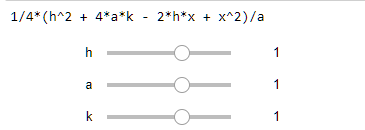I keep getting ValueError: Variable 'h' not found when I try to plot an interact:

    f = (x-h)^2 - 4*a*(y-k)
Parabola = solve(f,y).rhs()
print(Parabola)
@interact
def _(h=slider(-5,5,default=1), a=slider(-5,5,default=1), k=slider(-5,5,default=1)):
show(plot(Parabola,x,-5,5))


It even creates the sliders but the plot can't see h. What am I doing wrong? print(Parabola)edit retag close merge delete

Sort by » oldest newest most votedThis works for me:

var("a,h,k,x,y")
f = (x-h)^2 - 4*a*(y-k)
Parabola = y.subs(solve(f,y))
print(Parabola)
@interact
def _(h=slider(-5,5,default=1), a=slider(-5,5,default=1),
k=slider(-5,5,default=1)):
if a==0:
print("Invalid value for a. Please, select another value")
else:
show(plot(Parabola(a=a,h=h,k=k),x,-5,5))


Parabola is a symbolic expression which depends on x, but also on a, h and k. You must explicitly substitute the values of these variables in Parabola before plotting it as a function of x. Please, also note the alternative way to define Parabola and the caution that should be taken in the case a=0.

more